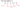## Identity Matrix

The identity matrix, when multiplied by any matrix of the same dimensions, results in the original matrix. It's just like multiplying numbers by 1. The identity matrix simply has 1's on the diagonal (upper left to lower right diagonal) and 0's elsewhere.

$\begin{bmatrix} 1 & 0 & 0 \\ 0 & 1 & 0 \\ 0 & 0 & 1 \end{bmatrix}$

When multiplying the identity matrix after some matrix $(A*I)$, the square identity matrix should match the other matrix's columns. When multiplying the identity matrix before some other matrix $(I*A)$, the square identity matrix should match the other matrix's rows.